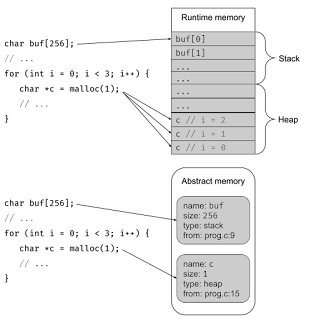## Thursday, February 23, 2023

### Reverse Engineering Notes - Stack Calling Convention

Stack Convention Basics

Stack diagram conventions refer to the way information is represented and organized in a stack data structure. A stack is a last-in, first-out (LIFO) data structure where elements are added and removed from the top of the stack. In a stack diagram, the elements in the stack are represented as a series of boxes stacked on top of each other, with the top of the stack at the highest position.

The workflow of the stack in a program can be described as follows:

1. When a function is called, a new stack frame is created and added to the top of the stack. The stack frame contains the parameters passed to the function, as well as any local variables declared within the function.

2. As the function executes, local variables are pushed onto the stack frame and popped off the stack frame as they are no longer needed. For example, if a variable is declared within a loop, it will be pushed onto the stack at the beginning of each iteration and popped off the stack at the end of the iteration.

3. When a function returns, its stack frame is removed from the top of the stack, and control returns to the calling function. The return value of the function is often stored in a register or memory location specified by the calling convention.

4. The process of adding and removing stack frames continues as functions are called and return until the program completes.

Here is an example of a stack diagram for a simple C program:

`c`
``````#include <stdio.h>

int factorial(int n) {
if (n <= 1) {
return 1;
} else {
return n * factorial(n-1);
}
}

int main() {
int x = 5;
int result = factorial(x);
printf("The factorial of %d is %d\n", x, result);
return 0;
}
``````

In this program, the `factorial()` function calculates the factorial of an integer using recursion. The `main()` function calls `factorial()` with an argument of 5 and prints the result. Here is the corresponding stack diagram for the program:

`lua`
``````|-----------------|         |-----------------|
|     factorial() |  ---->  |     factorial() |
|-----------------|         |-----------------|
|        n = 5    |         |        n = 4    |
|-----------------|         |-----------------|
|                 |         |                 |
|-----------------|         |-----------------|
|                 |         |     return 4    |
|-----------------|         |-----------------|
|                 |         |                 |
|-----------------|         |-----------------|
|                 |         |     factorial() |
|-----------------|         |-----------------|
|                 |         |        n = 3    |
|-----------------|         |-----------------|
|                 |         |                 |
|-----------------|         |-----------------|
|                 |         |     return 3    |
|-----------------|         |-----------------|
|                 |         |                 |
|-----------------|         |     factorial() |
|-----------------|         |-----------------|
|                 |         |        n = 2    |
|-----------------|         |-----------------|
|                 |         |                 |
|-----------------|         |-----------------|
|                 |         |     return 2    |
|-----------------|         |-----------------|
|                 |         |                 |
|-----------------|         |     factorial() |
|-----------------|         |-----------------|
|                 |         |        n = 1    |
|-----------------|         |-----------------|
|                 |         |                 |
|-----------------|         |     return 1    |
|-----------------|
``````